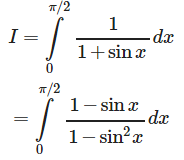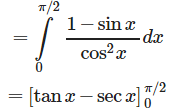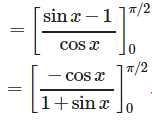# Test: Integrals (CBSE Level) - 1

## 25 Questions MCQ Test Mathematics (Maths) Class 12 | Test: Integrals (CBSE Level) - 1

Description
This mock test of Test: Integrals (CBSE Level) - 1 for JEE helps you for every JEE entrance exam. This contains 25 Multiple Choice Questions for JEE Test: Integrals (CBSE Level) - 1 (mcq) to study with solutions a complete question bank. The solved questions answers in this Test: Integrals (CBSE Level) - 1 quiz give you a good mix of easy questions and tough questions. JEE students definitely take this Test: Integrals (CBSE Level) - 1 exercise for a better result in the exam. You can find other Test: Integrals (CBSE Level) - 1 extra questions, long questions & short questions for JEE on EduRev as well by searching above.
QUESTION: 1

###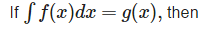Solution: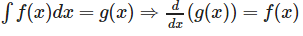QUESTION: 2

### Find the distance travelled by a car moving with acceleration given by a(t)=Sin(t), if it moves from t = 0 sec to t = π/2 sec, if velocity of a car at t=0sec is 10 km/hr.

Solution:

We know that,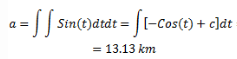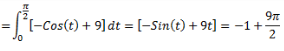QUESTION: 3

###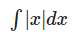Solution: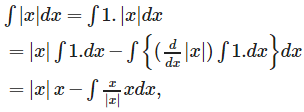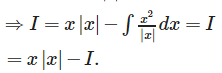QUESTION: 4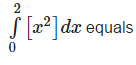Solution: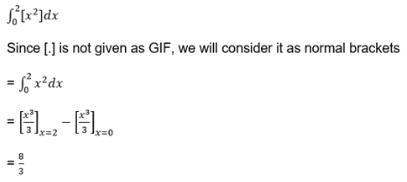QUESTION: 5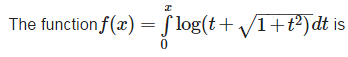Solution: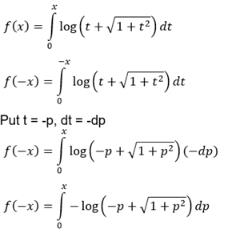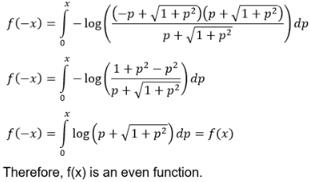QUESTION: 6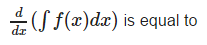Solution: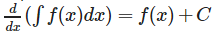QUESTION: 7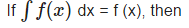Solution: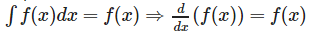QUESTION: 8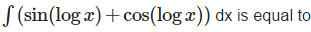Solution: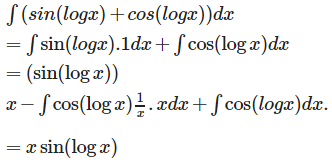QUESTION: 9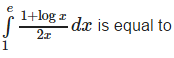Solution: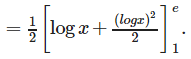QUESTION: 10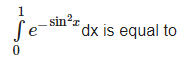Solution: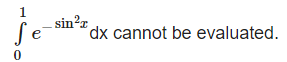QUESTION: 11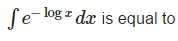Solution: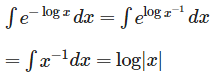QUESTION: 12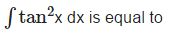Solution: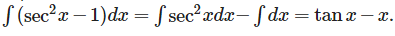QUESTION: 13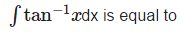Solution: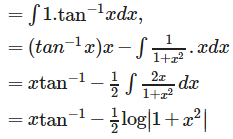QUESTION: 14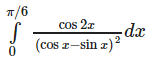Solution: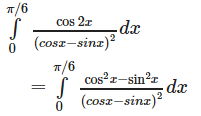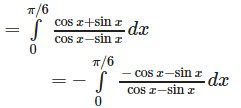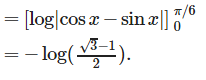QUESTION: 15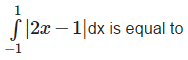Solution: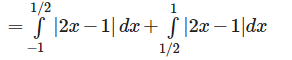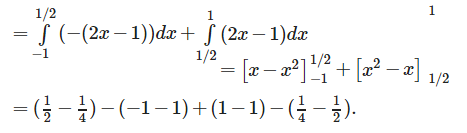QUESTION: 16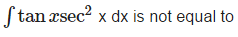Solution: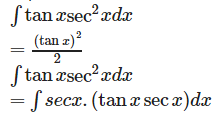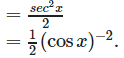Therefore the correct option is none of these.

QUESTION: 17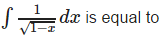Solution: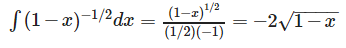QUESTION: 18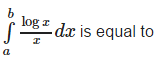Solution: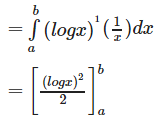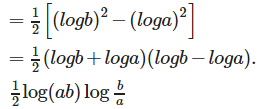QUESTION: 19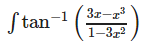dx can be evaluated by the substitution

Solution: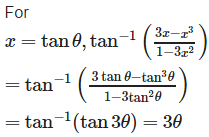QUESTION: 20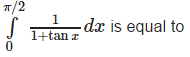Solution: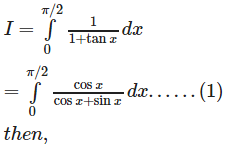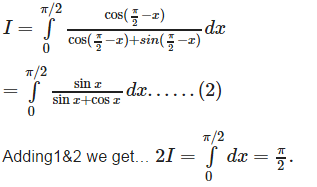QUESTION: 21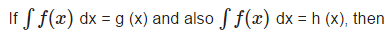Solution:

Since g(x) and h(x) are integrals of the same function , therefore ; g(x) – h(x) is constant.

QUESTION: 22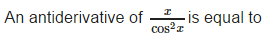Solution: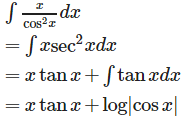QUESTION: 23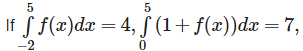then the value of the integral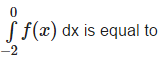Solution: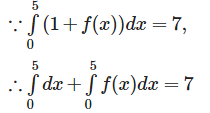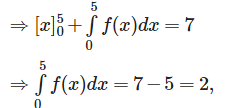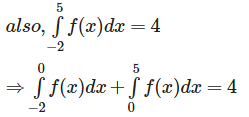QUESTION: 24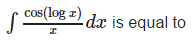Solution: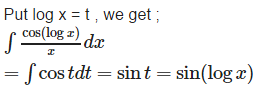QUESTION: 25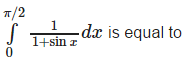Solution: